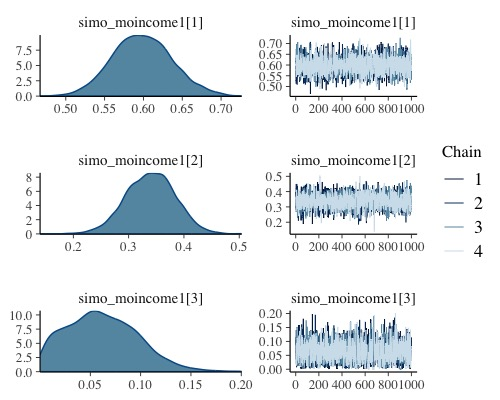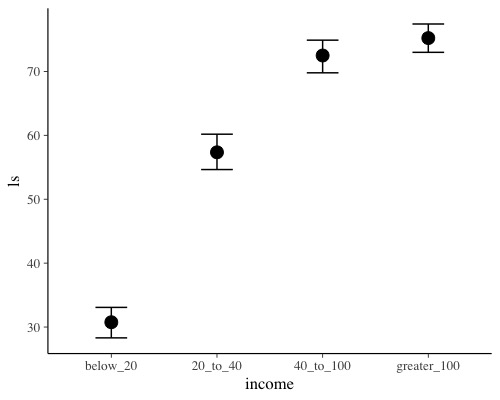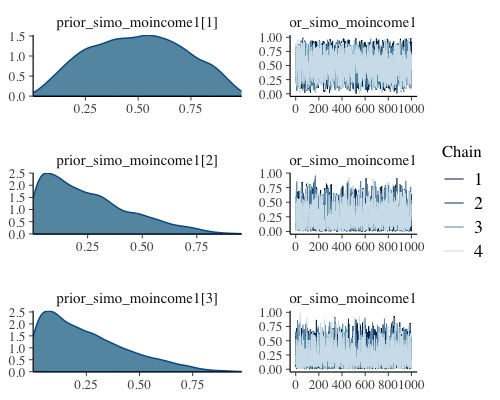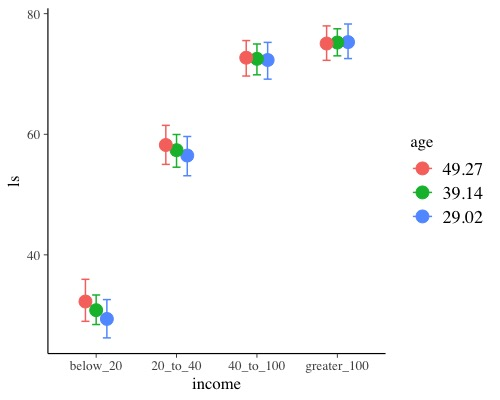# Estimating Monotonic Effects with brms

## Introduction

This vignette is about monotonic effects, a special way of handling discrete predictors that are on an ordinal or higher scale (Bürkner & Charpentier, in review). A predictor, which we want to model as monotonic (i.e., having a monotonically increasing or decreasing relationship with the response), must either be integer valued or an ordered factor. As opposed to a continuous predictor, predictor categories (or integers) are not assumed to be equidistant with respect to their effect on the response variable. Instead, the distance between adjacent predictor categories (or integers) is estimated from the data and may vary across categories. This is realized by parameterizing as follows: One parameter, $$b$$, takes care of the direction and size of the effect similar to an ordinary regression parameter. If the monotonic effect is used in a linear model, $$b$$ can be interpreted as the expected average difference between two adjacent categories of the ordinal predictor. An additional parameter vector, $$\zeta$$, estimates the normalized distances between consecutive predictor categories which thus defines the shape of the monotonic effect. For a single monotonic predictor, $$x$$, the linear predictor term of observation $$n$$ looks as follows:

$\eta_n = b D \sum_{i = 1}^{x_n} \zeta_i$

The parameter $$b$$ can take on any real value, while $$\zeta$$ is a simplex, which means that it satisfies $$\zeta_i \in [0,1]$$ and $$\sum_{i = 1}^D \zeta_i = 1$$ with $$D$$ being the number of elements of $$\zeta$$. Equivalently, $$D$$ is the number of categories (or highest integer in the data) minus 1, since we start counting categories from zero to simplify the notation.

## A Simple Monotonic Model

A main application of monotonic effects are ordinal predictors that can be modeled this way without falsely treating them either as continuous or as unordered categorical predictors. In Psychology, for instance, this kind of data is omnipresent in the form of Likert scale items, which are often treated as being continuous for convenience without ever testing this assumption. As an example, suppose we are interested in the relationship of yearly income (in $) and life satisfaction measured on an arbitrary scale from 0 to 100. Usually, people are not asked for the exact income. Instead, they are asked to rank themselves in one of certain classes, say: ‘below 20k’, ‘between 20k and 40k’, ‘between 40k and 100k’ and ‘above 100k’. We use some simulated data for illustration purposes. income_options <- c("below_20", "20_to_40", "40_to_100", "greater_100") income <- factor(sample(income_options, 100, TRUE), levels = income_options, ordered = TRUE) mean_ls <- c(30, 60, 70, 75) ls <- mean_ls[income] + rnorm(100, sd = 7) dat <- data.frame(income, ls) We now proceed with analyzing the data modeling income as a monotonic effect. fit1 <- brm(ls ~ mo(income), data = dat) The summary methods yield summary(fit1)  Family: gaussian Links: mu = identity; sigma = identity Formula: ls ~ mo(income) Data: dat (Number of observations: 100) Draws: 4 chains, each with iter = 2000; warmup = 1000; thin = 1; total post-warmup draws = 4000 Population-Level Effects: Estimate Est.Error l-95% CI u-95% CI Rhat Bulk_ESS Tail_ESS Intercept 30.72 1.22 28.31 33.08 1.00 2514 2623 moincome 14.84 0.56 13.77 15.94 1.00 2273 2416 Simplex Parameters: Estimate Est.Error l-95% CI u-95% CI Rhat Bulk_ESS Tail_ESS moincome1 0.60 0.04 0.53 0.68 1.00 2908 2493 moincome1 0.34 0.04 0.25 0.42 1.00 3253 2941 moincome1 0.06 0.04 0.00 0.14 1.00 1646 973 Family Specific Parameters: Estimate Est.Error l-95% CI u-95% CI Rhat Bulk_ESS Tail_ESS sigma 6.47 0.48 5.62 7.50 1.00 2553 2505 Draws were sampled using sampling(NUTS). For each parameter, Bulk_ESS and Tail_ESS are effective sample size measures, and Rhat is the potential scale reduction factor on split chains (at convergence, Rhat = 1). plot(fit1, variable = "simo", regex = TRUE)plot(conditional_effects(fit1))The distributions of the simplex parameter of income, as shown in the plot method, demonstrate that the largest difference (about 70% of the difference between minimum and maximum category) is between the first two categories. Now, let’s compare of monotonic model with two common alternative models. (a) Assume income to be continuous: dat$income_num <- as.numeric(dat$income) fit2 <- brm(ls ~ income_num, data = dat) summary(fit2)  Family: gaussian Links: mu = identity; sigma = identity Formula: ls ~ income_num Data: dat (Number of observations: 100) Draws: 4 chains, each with iter = 2000; warmup = 1000; thin = 1; total post-warmup draws = 4000 Population-Level Effects: Estimate Est.Error l-95% CI u-95% CI Rhat Bulk_ESS Tail_ESS Intercept 20.80 2.09 16.75 24.94 1.00 4150 2872 income_num 14.82 0.75 13.38 16.32 1.00 3873 2881 Family Specific Parameters: Estimate Est.Error l-95% CI u-95% CI Rhat Bulk_ESS Tail_ESS sigma 8.84 0.65 7.69 10.25 1.00 3793 2896 Draws were sampled using sampling(NUTS). For each parameter, Bulk_ESS and Tail_ESS are effective sample size measures, and Rhat is the potential scale reduction factor on split chains (at convergence, Rhat = 1). or (b) Assume income to be an unordered factor: contrasts(dat$income) <- contr.treatment(4)
fit3 <- brm(ls ~ income, data = dat)
summary(fit3)
 Family: gaussian
Links: mu = identity; sigma = identity
Formula: ls ~ income
Data: dat (Number of observations: 100)
Draws: 4 chains, each with iter = 2000; warmup = 1000; thin = 1;
total post-warmup draws = 4000

Population-Level Effects:
Estimate Est.Error l-95% CI u-95% CI Rhat Bulk_ESS Tail_ESS
Intercept    30.59      1.20    28.25    32.99 1.00     3088     2509
income2      26.76      1.87    23.01    30.42 1.00     3693     2789
income3      42.07      1.86    38.45    45.59 1.00     2887     2168
income4      44.57      1.68    41.31    47.86 1.00     3277     2925

Family Specific Parameters:
Estimate Est.Error l-95% CI u-95% CI Rhat Bulk_ESS Tail_ESS
sigma     6.47      0.48     5.62     7.51 1.00     3630     2834

Draws were sampled using sampling(NUTS). For each parameter, Bulk_ESS
and Tail_ESS are effective sample size measures, and Rhat is the potential
scale reduction factor on split chains (at convergence, Rhat = 1).

We can easily compare the fit of the three models using leave-one-out cross-validation.

loo(fit1, fit2, fit3)
Output of model 'fit1':

Computed from 4000 by 100 log-likelihood matrix

Estimate   SE
elpd_loo   -330.3  6.7
p_loo         4.6  0.7
looic       660.5 13.4
------
Monte Carlo SE of elpd_loo is 0.0.

All Pareto k estimates are good (k < 0.5).
See help('pareto-k-diagnostic') for details.

Output of model 'fit2':

Computed from 4000 by 100 log-likelihood matrix

Estimate   SE
elpd_loo   -360.5  6.0
p_loo         2.6  0.4
looic       721.1 12.0
------
Monte Carlo SE of elpd_loo is 0.0.

All Pareto k estimates are good (k < 0.5).
See help('pareto-k-diagnostic') for details.

Output of model 'fit3':

Computed from 4000 by 100 log-likelihood matrix

Estimate   SE
elpd_loo   -330.4  6.7
p_loo         4.7  0.7
looic       660.8 13.4
------
Monte Carlo SE of elpd_loo is 0.0.

All Pareto k estimates are good (k < 0.5).
See help('pareto-k-diagnostic') for details.

Model comparisons:
elpd_diff se_diff
fit1   0.0       0.0
fit3  -0.1       0.2
fit2 -30.3       5.9  

The monotonic model fits better than the continuous model, which is not surprising given that the relationship between income and ls is non-linear. The monotonic and the unordered factor model have almost identical fit in this example, but this may not be the case for other data sets.

## Setting Prior Distributions

In the previous monotonic model, we have implicitly assumed that all differences between adjacent categories were a-priori the same, or formulated correctly, had the same prior distribution. In the following, we want to show how to change this assumption. The canonical prior distribution of a simplex parameter is the Dirichlet distribution, a multivariate generalization of the beta distribution. It is non-zero for all valid simplexes (i.e., $$\zeta_i \in [0,1]$$ and $$\sum_{i = 1}^D \zeta_i = 1$$) and zero otherwise. The Dirichlet prior has a single parameter $$\alpha$$ of the same length as $$\zeta$$. The higher $$\alpha_i$$ the higher the a-priori probability of higher values of $$\zeta_i$$. Suppose that, before looking at the data, we expected that the same amount of additional money matters more for people who generally have less money. This translates into a higher a-priori values of $$\zeta_1$$ (difference between ‘below_20’ and ‘20_to_40’) and hence into higher values of $$\alpha_1$$. We choose $$\alpha_1 = 2$$ and $$\alpha_2 = \alpha_3 = 1$$, the latter being the default value of $$\alpha$$. To fit the model we write:

prior4 <- prior(dirichlet(c(2, 1, 1)), class = "simo", coef = "moincome1")
fit4 <- brm(ls ~ mo(income), data = dat,
prior = prior4, sample_prior = TRUE)

The 1 at the end of "moincome1" may appear strange when first working with monotonic effects. However, it is necessary as one monotonic term may be associated with multiple simplex parameters, if interactions of multiple monotonic variables are included in the model.

summary(fit4)
 Family: gaussian
Links: mu = identity; sigma = identity
Formula: ls ~ mo(income)
Data: dat (Number of observations: 100)
Draws: 4 chains, each with iter = 2000; warmup = 1000; thin = 1;
total post-warmup draws = 4000

Population-Level Effects:
Estimate Est.Error l-95% CI u-95% CI Rhat Bulk_ESS Tail_ESS
Intercept    30.75      1.22    28.40    33.10 1.00     2267     2296
moincome     14.82      0.56    13.73    15.93 1.00     2301     2007

Simplex Parameters:
Estimate Est.Error l-95% CI u-95% CI Rhat Bulk_ESS Tail_ESS
moincome1     0.60      0.04     0.52     0.67 1.00     2683     2179
moincome1     0.34      0.04     0.25     0.43 1.00     2871     2532
moincome1     0.06      0.03     0.01     0.13 1.00     1912     1071

Family Specific Parameters:
Estimate Est.Error l-95% CI u-95% CI Rhat Bulk_ESS Tail_ESS
sigma     6.46      0.47     5.61     7.48 1.00     3074     2755

Draws were sampled using sampling(NUTS). For each parameter, Bulk_ESS
and Tail_ESS are effective sample size measures, and Rhat is the potential
scale reduction factor on split chains (at convergence, Rhat = 1).

We have used sample_prior = TRUE to also obtain draws from the prior distribution of simo_moincome1 so that we can visualized it.

plot(fit4, variable = "prior_simo", regex = TRUE, N = 3)As is visible in the plots, simo_moincome1 was a-priori on average twice as high as simo_moincome1 and simo_moincome1 as a result of setting $$\alpha_1$$ to 2.

dat$age <- rnorm(100, mean = 40, sd = 10) We are not only interested in the main effect of age but also in the interaction of income and age. Interactions with monotonic variables can be specified in the usual way using the * operator: fit5 <- brm(ls ~ mo(income)*age, data = dat) summary(fit5)  Family: gaussian Links: mu = identity; sigma = identity Formula: ls ~ mo(income) * age Data: dat (Number of observations: 100) Draws: 4 chains, each with iter = 2000; warmup = 1000; thin = 1; total post-warmup draws = 4000 Population-Level Effects: Estimate Est.Error l-95% CI u-95% CI Rhat Bulk_ESS Tail_ESS Intercept 25.22 4.51 15.92 33.56 1.00 1402 1926 age 0.14 0.11 -0.07 0.38 1.00 1356 2192 moincome 16.83 2.07 13.08 21.05 1.00 1100 1925 moincome:age -0.05 0.05 -0.16 0.04 1.00 1085 1962 Simplex Parameters: Estimate Est.Error l-95% CI u-95% CI Rhat Bulk_ESS Tail_ESS moincome1 0.57 0.05 0.46 0.67 1.00 1637 2168 moincome1 0.35 0.06 0.24 0.47 1.00 2240 2264 moincome1 0.08 0.04 0.01 0.17 1.00 1852 1291 moincome:age1 0.35 0.24 0.01 0.85 1.00 2061 2287 moincome:age1 0.39 0.25 0.02 0.88 1.00 2055 2209 moincome:age1 0.26 0.21 0.01 0.76 1.00 2463 1795 Family Specific Parameters: Estimate Est.Error l-95% CI u-95% CI Rhat Bulk_ESS Tail_ESS sigma 6.45 0.48 5.58 7.49 1.00 3220 2563 Draws were sampled using sampling(NUTS). For each parameter, Bulk_ESS and Tail_ESS are effective sample size measures, and Rhat is the potential scale reduction factor on split chains (at convergence, Rhat = 1). conditional_effects(fit5, "income:age")## Modelling Monotonic Group-Level Effects Suppose that the 100 people in our sample data were drawn from 10 different cities; 10 people per city. Thus, we add an identifier for city to the data and add some city-related variation to ls. dat$city <- rep(1:10, each = 10)
var_city <- rnorm(10, sd = 10)
dat$ls <- dat$ls + var_city[dat\$city]

With the following code, we fit a multilevel model assuming the intercept and the effect of income to vary by city:

fit6 <- brm(ls ~ mo(income)*age + (mo(income) | city), data = dat)
summary(fit6)
 Family: gaussian
Links: mu = identity; sigma = identity
Formula: ls ~ mo(income) * age + (mo(income) | city)
Data: dat (Number of observations: 100)
Draws: 4 chains, each with iter = 2000; warmup = 1000; thin = 1;
total post-warmup draws = 4000

Group-Level Effects:
~city (Number of levels: 10)
Estimate Est.Error l-95% CI u-95% CI Rhat Bulk_ESS Tail_ESS
sd(Intercept)               4.95      1.96     1.98     9.63 1.00     1101     1118
sd(moincome)                0.70      0.58     0.02     2.21 1.00     1215     1433
cor(Intercept,moincome)     0.02      0.55    -0.94     0.95 1.00     3179     2445

Population-Level Effects:
Estimate Est.Error l-95% CI u-95% CI Rhat Bulk_ESS Tail_ESS
Intercept       28.31      4.68    19.06    37.20 1.00     1087     1820
age              0.13      0.11    -0.07     0.35 1.00     1166     2028
moincome        16.28      2.00    12.64    20.32 1.00      985     1729
moincome:age    -0.04      0.05    -0.14     0.05 1.00      938     1718

Simplex Parameters:
Estimate Est.Error l-95% CI u-95% CI Rhat Bulk_ESS Tail_ESS
moincome1         0.57      0.05     0.46     0.67 1.00     1686     2206
moincome1         0.36      0.06     0.25     0.47 1.00     2534     2754
moincome1         0.07      0.04     0.01     0.17 1.00     2085     1120
moincome:age1     0.35      0.24     0.02     0.83 1.00     3103     2769
moincome:age1     0.38      0.25     0.01     0.88 1.00     2834     2601
moincome:age1     0.28      0.21     0.01     0.77 1.00     3291     2752

Family Specific Parameters:
Estimate Est.Error l-95% CI u-95% CI Rhat Bulk_ESS Tail_ESS
sigma     6.31      0.51     5.42     7.40 1.00     3454     1976

Draws were sampled using sampling(NUTS). For each parameter, Bulk_ESS
and Tail_ESS are effective sample size measures, and Rhat is the potential
scale reduction factor on split chains (at convergence, Rhat = 1).

reveals that the effect of income varies only little across cities. For the present data, this is not overly surprising given that, in the data simulations, we assumed income to have the same effect across cities.